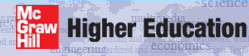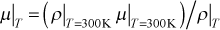# Institut für Thermodynamik der Luft- und Raumfahrt - Universität StuttgartMechanical Engineering - The University of Texas at Austin# fluid properties

In TEXSTAN the user can choose either a constant property solution or a variable property solution. If it is a constant property solution the thermal energy equation is considered a temperature equation, and if it a variable property solution the thermal energy equation is considered a stagnation enthalpy equation. The corresponding temperature field is then determined by a two-step process of reducing the stagnation enthalpy field to a static enthalpy field and then converting that field to the temperature field using the appropriate fluid static enthalpy-temperature relationship. Constant fluid properties are user-supplied via the input data set, and variable fluid properties are supplied through TEXSTAN subroutines, with limited user input for certain models.

In TEXSTAN the choice of whether to model a convective problem using constant or variable properties is primarily based on the ratio of the expected surface-to-mass-averaged temperature (mean temperature) for internal flows, or ratio of surface-to-free stream temperature for external flows, where the temperature is required to be absolute temperature (Kelvins or degrees Rankine). When this ratio falls outside the interval from 0.95 to 1.05, the use of variable properties should be considered, and especially if the fluid is liquid. Note that TEXSTAN does not require the use of variable properties to compute the effect of viscous work (or the effect of viscous dissipation), because this is an independent effect. For constant properties, the user supplies quantities such as density, dynamic viscosity, and Prandtl number.

The property tables and functions within TEXSTAN come from a variety of sources. The units on all properties conform to the units convention declared with the flag kunits, and described in overview: units.

kfluid type of fluid to be considered
=1 constant properties
>1 variable properties, requires solving energy equation
=13 air at low pressure (adapted from our textbook CHMT)
=4 water
=6 nitrogen
=8 helium
=14 air ideal gas, Pr=const, cp=const, Sutherland viscosity law
=15 air similar to kfluid=14 but sets (ρ*μ)=const
=16 motor oil (adapted from our textbook CHMT)

kfluid =13 is a table of entries for dry air in the temperature range 100-2500 K (or) 243-4500 °R. The effects of pressure on all properties except density are ignored. Air data at the lowest temperature has been obtained via extrapolation from 135 K. Low temperature data are primarily from Sychev et al.1, and high temperature data is primarily from Hippensteele and Colladay2. This is the recommended property table for variable property air (replacing the kfluid=2 choice), and its values replicate CHMT.

kfluid =6 is a table of entries for dry nitrogen in the temperature range 180-1800 °R. (100-1000 K). The effects of pressure on all properties except density are ignored. Reference for the properties is Keenan and Kaye Gas Tables4, with viscosity and Pr from Touloukian et al.5.

kfluid =8 is a table of entries for dry helium in the temperature range 300-3200 ºR. (167-1778K). The effects of pressure on all properties except density are ignored. Reference for the properties is McCarty6.

The tables of properties for kfluid =2,6,8 are of the ideal gas model class, and the properties are all handled the same way. They are linearly interpolated. They contain static enthalpy (i), temperature (T), dynamic viscosity (μ), Prandtl number (Pr), and the ratio of specific heats (γ). The tables can be used interchangeably, such that the interpolation can be carried out as i(T) or as T(i). For example, the input variable stagnation temperature for the flow, (tstag) is easily converted to stagnation enthalpy. Another important example is the initial profile for the stagnation enthalpy equation. The profile is first created as a static temperature profile, then converted to a static enthalpy profile using the property routine, and then the kinetic energy is added to create the stagnation enthalpy profile. The ideal gas law is used to calculate the density, ρ = P/(RT) and the Mach number, M = u /(γRT) 0.5, where R is the molecular-specific gas constant for the gas (equal to the universal gas constant divided by the molecular weight of the gas).

kfluid =4 is a set of correlations for saturated water between 273.15 K and 573.15 K (491.7-1031.7 °R). The reference for the water properties is ASHRAE3, which contain correlations for specific heat, density, and thermal conductivity as a function of temperature.

kfluid =14 is any gas that can be modeled using the perfect gas model. The required input variables will include γ, the ratio of specific heats (the gam/cp variable), the gas molecular weight (the amolwt variable), and the Prandtl number. For the perfect gas model the Prandtl number is required to be constant. The density is computed using the ideal gas law ρ = P/(RT). For the perfect gas model the specific heat is also required to be constant, and it is computed from the ideal gas law, cp = (Rγ)/(γ-1). The Mach number is computed using the ideal gas law, M = u / √(γRT). The viscosity is computed from the Sutherland law (same as used in kfluid=13). Note that in all these equations R is the molecular-specific gas constant rather than the universal gas constant.

This property table is NOT recommended for flows with significant property variation due to either large temperature differences or viscous dissipation because of the issue of what temperature to use to specify the Prandtl number and the ratio of specific heats.

kfluid =15 is a variation on kfluid=14, where the viscosity is computed such that the product (ρμ)=constant, leading tokfluid =16 a table of entries for oil as described in Table A-14 of Appendix A of our textbook CHMT for the temperature range 273-423 K (or) 491-761 °R. The properties are linearly interpolated.

## References

1
Sychev et al., Thermodynamic Properties of Air, T. B. Selover, Jr., ed., Hemisphere, Washington, 1987.
2
Hippensteele, S. A., and R. S. Colladay, Computer Program for Obtaining Thermodynamic and Transport Properties of Air and Products of Combustion of ASTM-A-a Fuel and Air, NASA TP 1160, 1978.
3
Thermophysical Properties of Refrigerants, ASHRAE (American Society of Heating, Refrigeration, and Air Conditioning), New York, 1976.
4
Keenan, J. H., J. Kaye, Gas Tables, John Wiley and Sons, New York, 1945.
5
Touloukian et al., Thermophysical Properties of Matter, IFI/Plenum, New York, 3,6,11, 1970.
6
McCarty, R. D., Thermophysical Properties of Helium-4 from 2-1500 K with Pressures to 1000 Atmospheres, NBS TN 631, 1972.

website updated Sept 2010   © 1996-2010 Michael E. Crawford - all rights reserved - website validated for CSS 2.1 and XHTML 1.0 strict at www.w3.org